# RD Sharma Class 9 Solutions Chapter 4 Algebraic Identities MCQS

## RD Sharma Class 9 Solutions Chapter 4 Algebraic Identities MCQS

These Solutions are part of RD Sharma Class 9 Solutions. Here we have given RD Sharma Class 9 Solutions Chapter 4 Algebraic Identities MCQS

Question 1.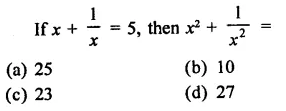Solution: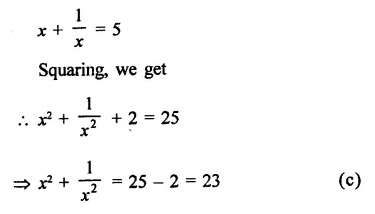Question 2.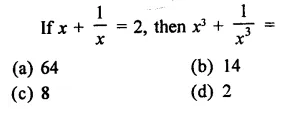Solution: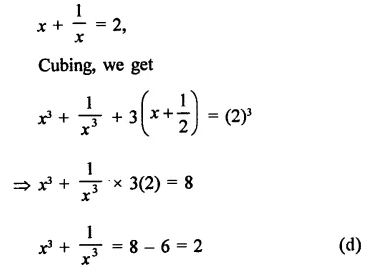Question 3.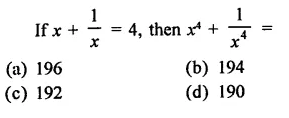Solution: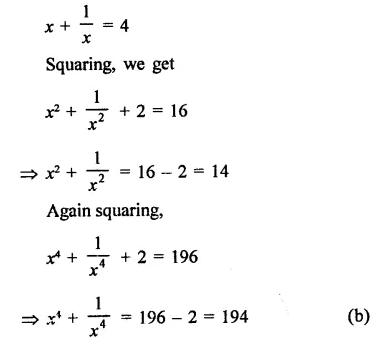Question 4.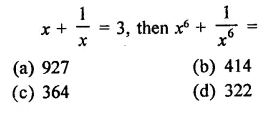Solution: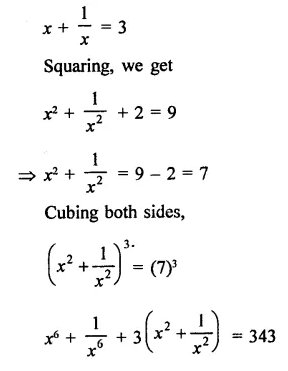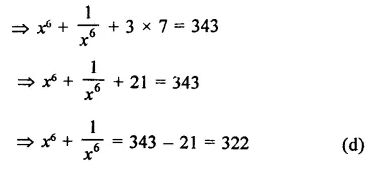Question 5.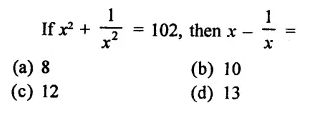Solution: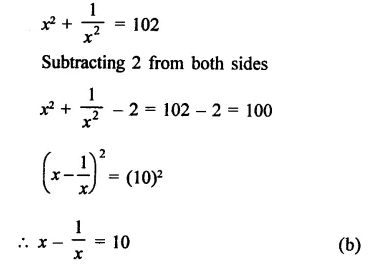Question 6.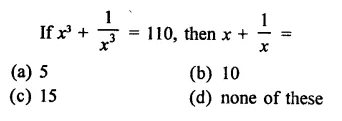Solution: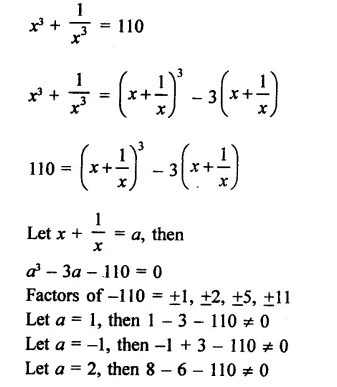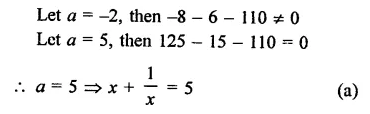Question 7.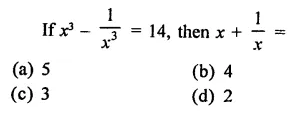Solution: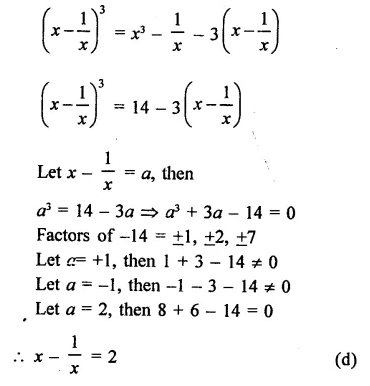Question 8.
If a + b + c = 9 and  ab + bc + ca = 23, then a2 + b2 + c2 =
(a) 35
(b) 58
(c) 127
(d) none of these
Solution:
a + b + c = 9, ab + bc + ca = 23
Squaring,
(a + b+ c) = (9)2
a2 + b2 + c2 + 2 (ab + bc + ca) = 81
⇒ a2 + b2 + c2 + 2 x 23 = 81
⇒  a2 + b2+ c2 + 46 = 81
⇒  a2 + b2+ c2 = 81 – 46 = 35   (a)

Question 9.
(a – b)3 + (b – c)3 + (c – a)3 =
(a) (a + b + c) (a2 + b2 + c2 – ab – bc – ca)
(d) (a -b)(b- c) (c – a)
(c) 3(a – b) (b – c) (c – a)
(d) none of these
Solution:
(a – b)3 + (b- c)3 + (c- a)3
∵ a – b + b – c + c – a = 0
∴ (ab)3 + (b – c)3 + (c – a)3
= 3
(a -b)(b- c) (c – a)        (c)

Question 10.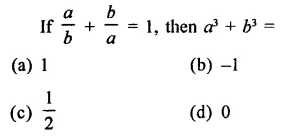Solution: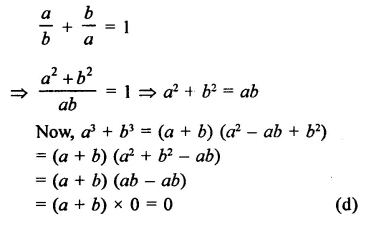Question 11.
If a – b = -8 and ab = -12 then a3 – b3 =
(a) -244
(b) -240
(c) -224
(d) -260
Solution:
a – b = -8, ab = -12
(a – b)3 = a3 – b3 – 3ab (a – b)
(-8)3 = a3 – b3 – 3 x (-12) (-8)
-512 = a3-b3– 288
a3 – b3 = -512 + 288 = -224      (c)

Question 12.
If the volume of a cuboid is 3x2 – 27, then its possible dimensions are
(a) 3, x2, -27x
(b) 3, x – 3, x + 3
(c) 3, x2, 27x
(d) 3, 3, 3
Solution:
Volume = 3x2 -27 = 3(x2 – 9)
= 3(x + 3) (x – 3)
∴ Dimensions are   = 3, x – 3, x   +  3        (b)

Question 13.
75 x 75 + 2 x 75 x 25    + 25 x 25 is equal to
(a) 10000
(b) 6250
(c) 7500
(d) 3750
Solution: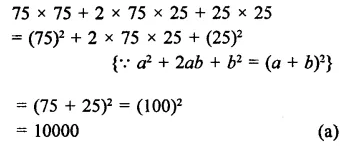Question 14.
(x – y) (x + y)(x2 + y2) (x4 + y4) is equal to
(a) x16 – y16
(b) x8 – y8
(c) x8 + y8
(d) x16 + y16
Solution: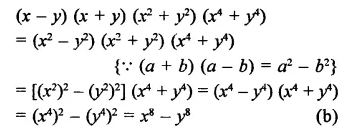Question 15.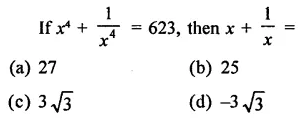Solution: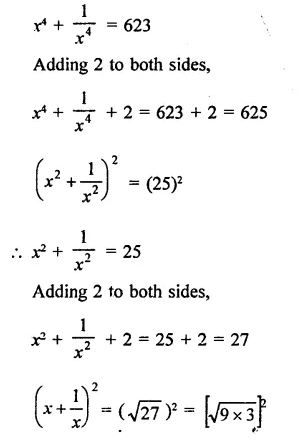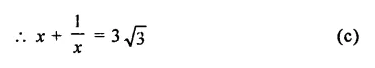Question 16.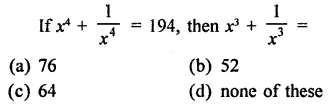Solution: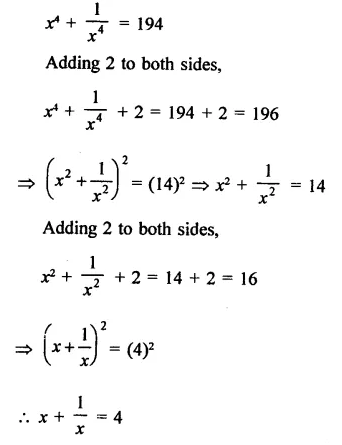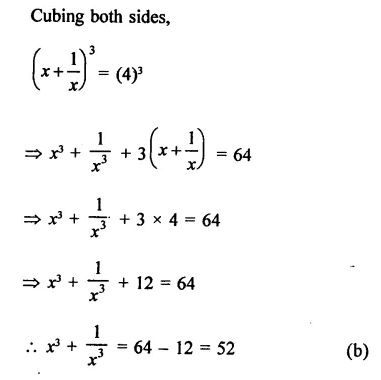Question 17.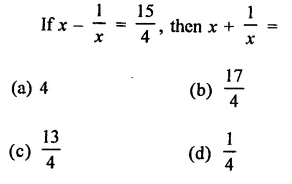Solution: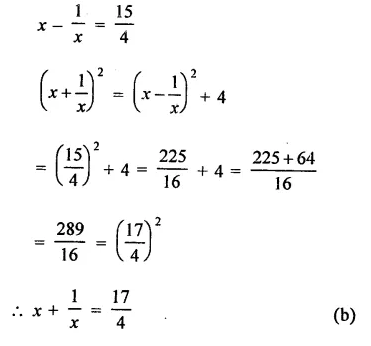Question 18.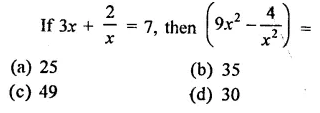Solution: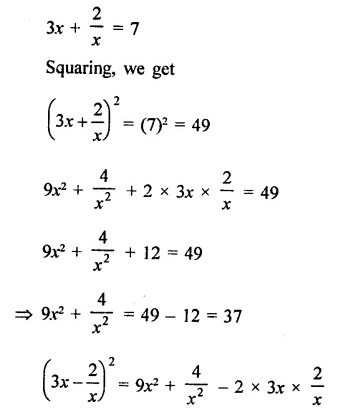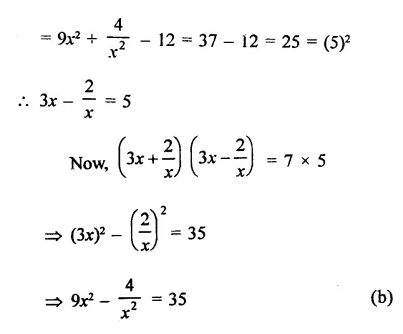Question 19.
If a2 + b2 + c2 – ab – bc – ca = 0, then
(a) a + b = c
(b) b + c = a
(c) c + a = b
(d) a = b = c
Solution:
a2 + b2 + c2 – ab – bc – ca = 0
2 {a2 + b2 + c2 – ab – be – ca) = 0 (Multiplying by 2)
⇒  2a2 + 2b2 + 2c2– 2ab – 2bc – 2ca = 0
⇒  a2 + b2 – 2ab + b2 + c2 – 2bc + c2 + a2 – 2ca = 0
⇒  (a – b)2 + (b – c)2 + (c – a)2 = 0
(a – b)2 = 0, then a – b = 0
⇒ a = b
Similarly, (b – c)2 = 0, then
b-c = 0
⇒ b = c
and (c – a)2 = 0, then c-a = 0
⇒ c = a
∴ a = b – c           (d)

Question 20.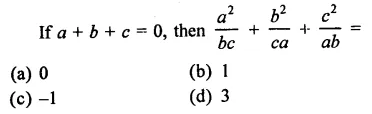Solution: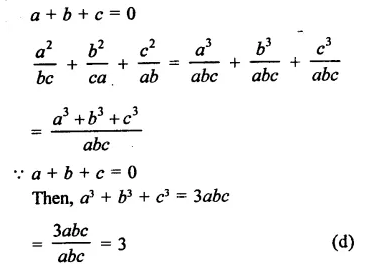Question 21.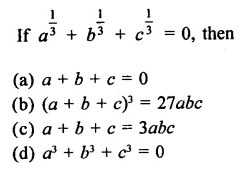Solution: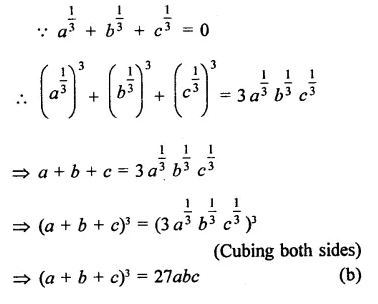Question 22.
If a + b + c = 9 and ab + bc + ca = 23, then a3 + b3 + c3 – 3 abc =
(a) 108
(b) 207
(c) 669
(d) 729
Solution:
a3 + b3 + c3 – 3abc
= (a + b + c) [a2 + b2 + c2 – (ab + bc + ca)
Now, a + b + c = 9
Squaring,
a2 + b2 + c2 + 2 (ab + be + ca) = 81
⇒  a2 + b2 + c2 + 2 x 23 = 81
⇒  a2 + b2 + c2 + 46 = 81
⇒  a2 + b2 + c2 = 81 – 46 = 35
Now, a3 + b3 + c3 – 3 abc = (a + b + c) [(a2 + b2 + c2) – (ab + bc + ca)
= 9[35 -23] = 9 x 12= 108                     (a)

Question 23.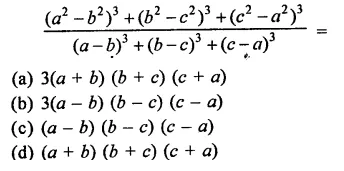Solution: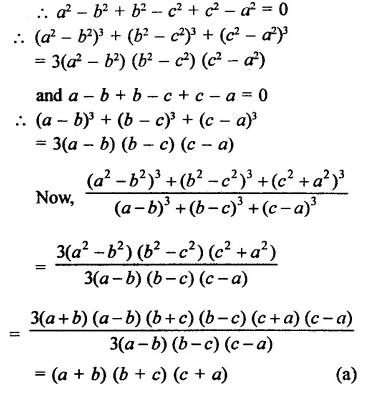Question 24.
The product (a + b) (a – b) (a2 – ab + b2) (a2 + ab + b2) is equal to
(a) a6 +   b6
(b) a6 – b6
(c) a3 – b3
(d) a3 + b3
Solution:
(a + b) (a – b) (a2 – ab + b2) (a2 + ab +b2)
= (a + b) (a2-ab + b2) (a-b) (a2 + ab + b2)
= (a3 + b3) (a3 – b3)
= (a3)2 – (b3)2 = a6 – b6   (b)

Question 25.
The product (x2 – 1) (x4 + x2 + 1) is equal to
(a) x8 –   1
(b) x8 + 1
(c) x6 –   1
(d) x6 + 1
Solution:
(x2 – 1) (x4 + x2 + 1)
= (x2)3 – (1)3 = x6 – 1                            (c)

Question 26.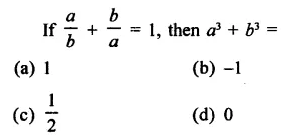Solution: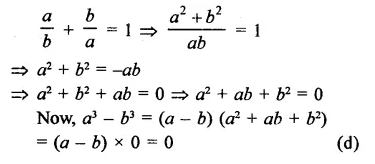Question 27.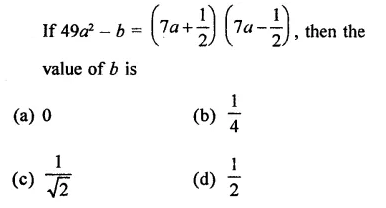Solution: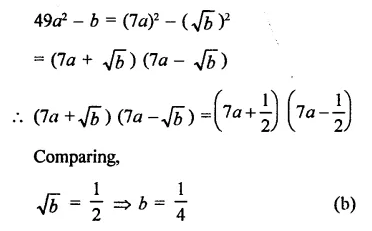Hope given RD Sharma Class 9 Solutions Chapter 4 Algebraic Identities MCQS are helpful to complete your math homework.

If you have any doubts, please comment below. Learn Insta try to provide online math tutoring for you.